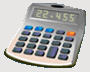DosageHelp.com Helping Nursing Students Prepare for Medication Exams By Explaining Dosage Calculations

# Volume/Time - IV mL Rate Questions

Given a certain amount of liquid and a time period, what is the necessary IV flow rate in mL/hr? Measurement used when IV regulated electronically by infusion pump.

Formula:
 Volume (mL) Time (hr)
=  Y (Flow Rate in mL/hr)

Example: Infuse 250 mL over the next 120 minutes by infusion pump.
 Volume (mL) Time (hr)
=  Y (Flow Rate in mL/hr)
Convert 120 minutes to hours.
• min → hr    ( ÷ by 60 )
• 120 min ÷ 60 = 2 hr
 250 mL 2 hr
=  125 mL/hr

Example: Ordered 1000 mL D5W IV to infuse in 10 hours by infusion pump.
 Volume (mL) Time (hr)
=  Y (Flow Rate in mL/hr)
 1000 mL 10 hr
=  100 mL/hr

Home Dosage Question Steps Unit Conversion Mass for Mass Mass/Liquid For Liquid IV Terms & Abbreviations Amount in IV Fluid Volume/Time - IV mL Rate Volume/Time - IV Drop Rate Fluid Maintenance Dosage By Weight Mass/Time - IV mL Rate Practice Questions Question of the Day Tell a Friend Print a Flyer